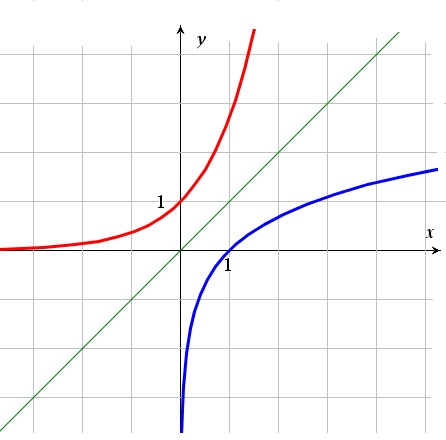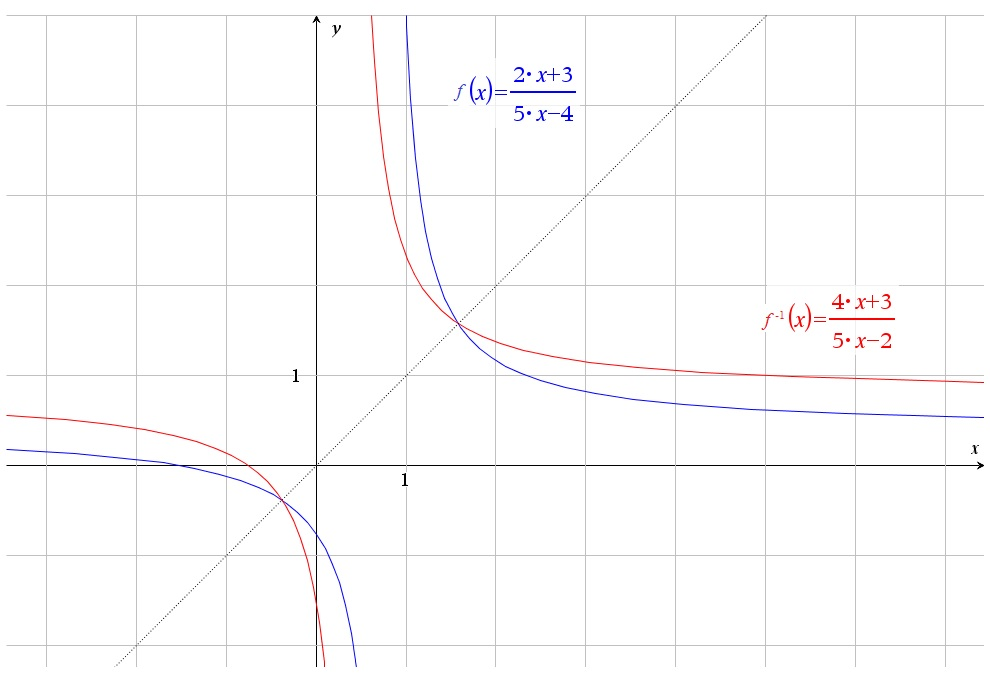# The Inverse of a function

Up a level : Algebra and Arithmetic
Previous page : Properties of Functions
Next page : Numbers, an introductionThe Inverse of a function

If  f : AB  is a bijective function, then, by the definition of a bijective function,  the mapping from B to A is also a function. This is the inverse function to f , or inverse of  f, mostly written f -1. The inverse of  f -1 will then be  f.

If the functions is an explicit function, y=f(x), then we can write its inverse as

x=f -1(y)

We usually though follow the convention that x is the independent variable, and thus write the inverse function as

y=f -1(x).

If a bijective mapping maps a to b, then its inverse maps b to a, this means that,

a=f -1(f (a)),

and,

b=f (f -1(b))

The function f(x)=3x, for example, has as inverse f(y)=x/3, and we have,

f (f -1(x))=f (x/3)=3x/3=x

and

f -1(f (x))=f -1(3x)=3x/3=x

Say a function maps a to b. That means that the set corresponding to the function would contain the element (a, b), and a graph of the function would have a point at that point – or rather the point (a, b) satisfies the function.  The inverse function will now contain the point (b, a). This means that if the domain and range are the reals, then the inversion of a function is a mirroring of the graph through the line y=x.This because we simply swap the coordinates of x and y.

Recipe for finding the inverse function

1. If the function is written in the the form f(x)=something, replace the f(x) with y.
2. Swap the x‘s and the y‘s.
3. Make y the subject in what you have now.
4. Finally, replace the y with f -1(x).

Let us for example take$f(x)=\frac{2x+3}{5x-4}$

1.$y=\frac{2x+3}{5x-4}$
1.$x=\frac{2y+3}{5y-4}$
1.  What we have: What we do:$x = \frac{{2y + 3}}{{5y - 4}}$$x = \frac{{2y + 3}}{{5y - 4}}$ //$x \cdot (5y - 4)$$x \cdot (5y - 4)$$x(5y - 4) = 2y + 3$$x(5y - 4) = 2y + 3$ //Expand.$5xy - 4x = 2y + 3$$5xy - 4x = 2y + 3$ //Collect all y‘s on one side: −2y$5xy - 4x - 2y = 3$$5xy - 4x - 2y = 3$ //All the rest on the other side: +4x$5xy - 2y = 4x + 3$$5xy - 2y = 4x + 3$ //Factorize the left hand side.$y(5x - 2) = \;4x + 3$$y(5x - 2) = \;4x + 3$ //$\div (5x - 2)$$\div (5x - 2)$$y = \frac{{4x + 3\;}}{{5x - 2}}$$y = \frac{{4x + 3\;}}{{5x - 2}}$
2.${f^{ - 1}}(x) = \frac{{4x + 3\;}}{{5x - 2}}$

If we test with x=10 we get$f(10)=\frac{2\cdot 10+3}{5\cdot 10-4}=\frac{23}{46}=\frac{1}{2}$

Now having this as the input to the inverse function will give us${{f}^{-1}}\left( \frac{1}{2} \right)=\frac{4\cdot \frac{1}{2}+3\ }{5\cdot \frac{1}{2}-2}=\frac{4+3\cdot 2\ }{5-2\cdot 2}=\frac{10}{1}=10$

I.e. we get 10 back, as expected.

If we graph these two functions (and y=x) we can see that they are reflections along the y=x – axis .Up a level : Algebra and Arithmetic
Previous page : Properties of Functions
Next page : Numbers, an introductionLast modified: Jan 13, 2017 @ 17:26